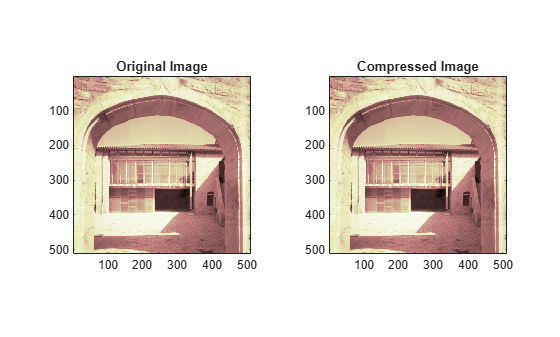## 2-D Discrete Wavelet Analysis

This section takes you through the features of 2-D discrete wavelet analysis using the Wavelet Toolbox™ software. The toolbox provides these functions for image analysis. For more information, see the function reference pages.

Note

In this section the presentation and examples use 2-D arrays corresponding to indexed image representations. However, the functions described are also available when using truecolor images, which are represented by `m`-by-`n`-by-3 arrays of `uint8`.

### Analysis-Decomposition Functions

Function Name

Purpose

`dwt2`

Single-level decomposition

`wavedec2`

Decomposition

`wmaxlev`

Maximum wavelet decomposition level

### Synthesis-Reconstruction Functions

Function Name

Purpose

`idwt2`

Single-level reconstruction

`waverec2`

Full reconstruction

`wrcoef2`

Selective reconstruction

`upcoef2`

Single reconstruction

### Decomposition Structure Utilities

Function Name

Purpose

`detcoef2`

Extraction of detail coefficients

`appcoef2`

Extraction of approximation coefficients

`upwlev2`

Recomposition of decomposition structure

### Denoising and Compression

Function Name

Purpose

`wdenoise2`

Wavelet image denoising

`ddencmp`

Provide default values for denoising and compression

`wbmpen`

Penalized threshold for wavelet 1-D or 2-D denoising

`wdcbm2`

Thresholds for wavelet 2-D using Birgé-Massart strategy

`wdencmp`

Wavelet denoising and compression

`wthrmngr`

Threshold settings manager

In this section, you'll learn

• How to load an image

• How to analyze an image

• How to compress an image

### Wavelet Image Analysis and Compression

This example shows how you can use 2-D wavelet analysis to compress an image efficiently without sacrificing its clarity.

Note: Instead of directly using `image(I)` to visualize the image `I`, we use `image(wcodemat(I))`, which displays a rescaled version of `I` leading to a clearer presentation of the details and approximations (see `wcodemat`).

```load wbarb whos X map```
``` Name Size Bytes Class Attributes X 256x256 524288 double map 192x3 4608 double ```

Display the image.

```image(X) colormap(map) colorbar```If the colormap is smooth, the wavelet transform can be directly applied to the indexed image; otherwise the indexed image should be converted to grayscale format. For more information, see Wavelets: Working with Images. Since the colormap is smooth in this image, you can now perform the decomposition.

Perform a single-level wavelet decomposition of the image using the `bior3.7` wavelet. The coefficient matrix `cA1` are the approximation coefficients. The horizontal, vertical, and diagonal details are in the matrices `cH1`, `cV1`, and `cD1`, respectively.

```wv = 'bior3.7'; [cA1,cH1,cV1,cD1] = dwt2(X,wv);```

Use `idwt1` to construct the approximations and details from the coefficients. (Note: You can also use `upcoef2`.

```sx = size(X); A1 = idwt2(cA1,[],[],[],wv,sx); H1 = idwt2([],cH1,[],[],wv,sx); V1 = idwt2([],[],cV1,[],wv,sx); D1 = idwt2([],[],[],cD1,wv,sx);```

Display the approximations and details.

```figure subplot(2,2,1) image(wcodemat(A1,192)) title('Approximation A1') subplot(2,2,2) image(wcodemat(H1,192)) title('Horizontal Detail H1') subplot(2,2,3) image(wcodemat(V1,192)) title('Vertical Detail V1') subplot(2,2,4) image(wcodemat(D1,192)) title('Diagonal Detail D1') colormap(map)```Regenerate the image by the single-level inverse discrete wavelet transform. Confirm the difference between the regenerated and original images are small.

```Xrec = idwt2(cA1,cH1,cV1,cD1,wv); max(abs(X(:)-Xrec(:)))```
```ans = 1.4211e-13 ```

Perform a level-2 wavelet decomposition of the image using the same `bior3.7` wavelet. The coefficients of all the components of a second-level decomposition (that is, the second-level approximation and the first two levels of detail) are returned concatenated into one vector, `C`. Argument `S` is a bookkeeping matrix that keeps track of the sizes of each component.

`[c,s] = wavedec2(X,2,wv);`

Extract the level 2 approximation coefficients. Extract the first- and second-level detail coefficients.

```cA2 = appcoef2(c,s,wv,2); [cH2,cV2,cD2] = detcoef2('all',c,s,2); [cH1,cV1,cD1] = detcoef2('all',c,s,1);```

Reconstruct the level 2 approximation and the level 1 and level 2 details.

```A2 = wrcoef2('a',c,s,wv,2); H1 = wrcoef2('h',c,s,wv,1); V1 = wrcoef2('v',c,s,wv,1); D1 = wrcoef2('d',c,s,wv,1); H2 = wrcoef2('h',c,s,wv,2); V2 = wrcoef2('v',c,s,wv,2); D2 = wrcoef2('d',c,s,wv,2);```

Display the approximation and details.

```figure subplot(2,4,1) image(wcodemat(A1,192)) title('Approximation A1') subplot(2,4,2) image(wcodemat(H1,192)) title('Horizontal Detail H1') subplot(2,4,3) image(wcodemat(V1,192)) title('Vertical Detail V1') subplot(2,4,4) image(wcodemat(D1,192)) title('Diagonal Detail D1') subplot(2,4,5) image(wcodemat(A2,192)) title('Approximation A2') subplot(2,4,6) image(wcodemat(H2,192)) title('Horizontal Detail H2') subplot(2,4,7) image(wcodemat(V2,192)) title('Vertical Detail V2') subplot(2,4,8) image(wcodemat(D2,192)) title('Diagonal Detail D2') colormap(map)```Compress the image. Use `ddencmp` to calculate the default parameters and `wdencmp` to perform the actual compression.

```[thr,sorh,keepapp] = ddencmp('cmp','wv',X); [Xcomp,CXC,LXC,PERF0,PERFL2] = ... wdencmp('gbl',c,s,wv,2,thr,sorh,keepapp);```

Compare the compressed image with the original image.

```fprintf('Percentage of wavelet coefficients set to zero: %.4f\nPercentage of energy preserved: %.4f\n',... PERF0,PERFL2);```
```Percentage of wavelet coefficients set to zero: 49.8011 Percentage of energy preserved: 99.9817 ```
```figure subplot(121) image(X) title('Original Image') axis square subplot(122) image(Xcomp) title('Compressed Image') axis square colormap(map)```Note that, even though the compressed image is constructed from only about half as many nonzero wavelet coefficients as the original, there is almost no detectable deterioration in the image quality.